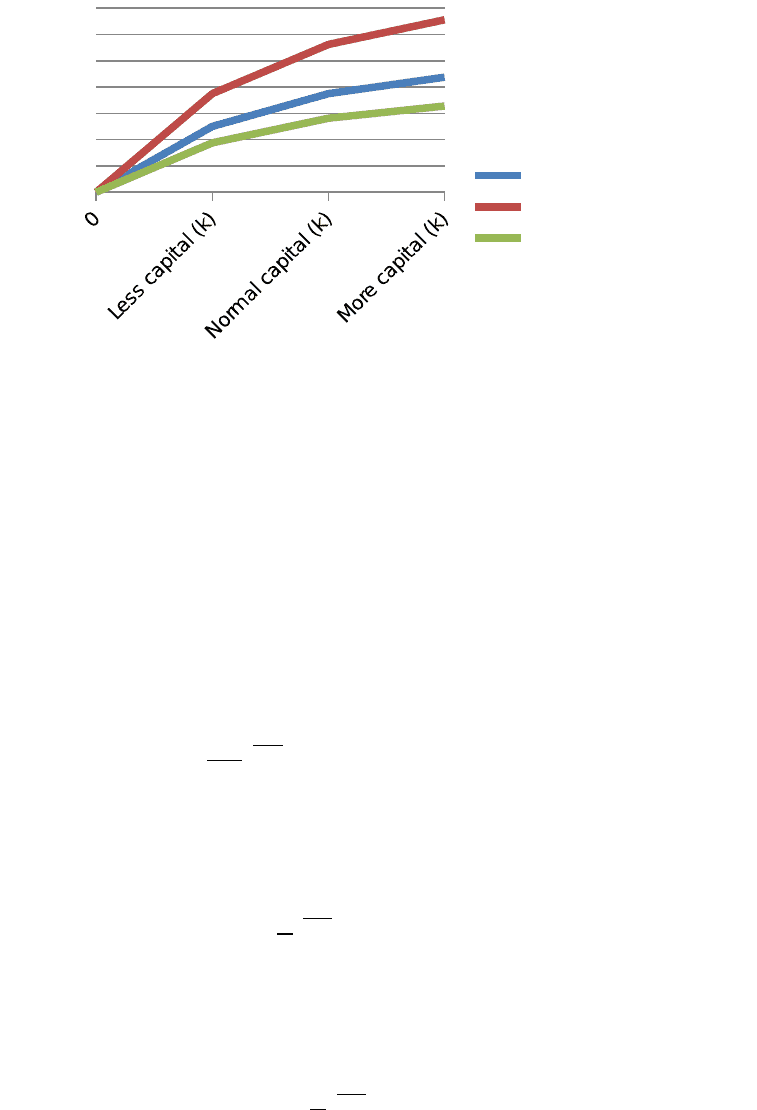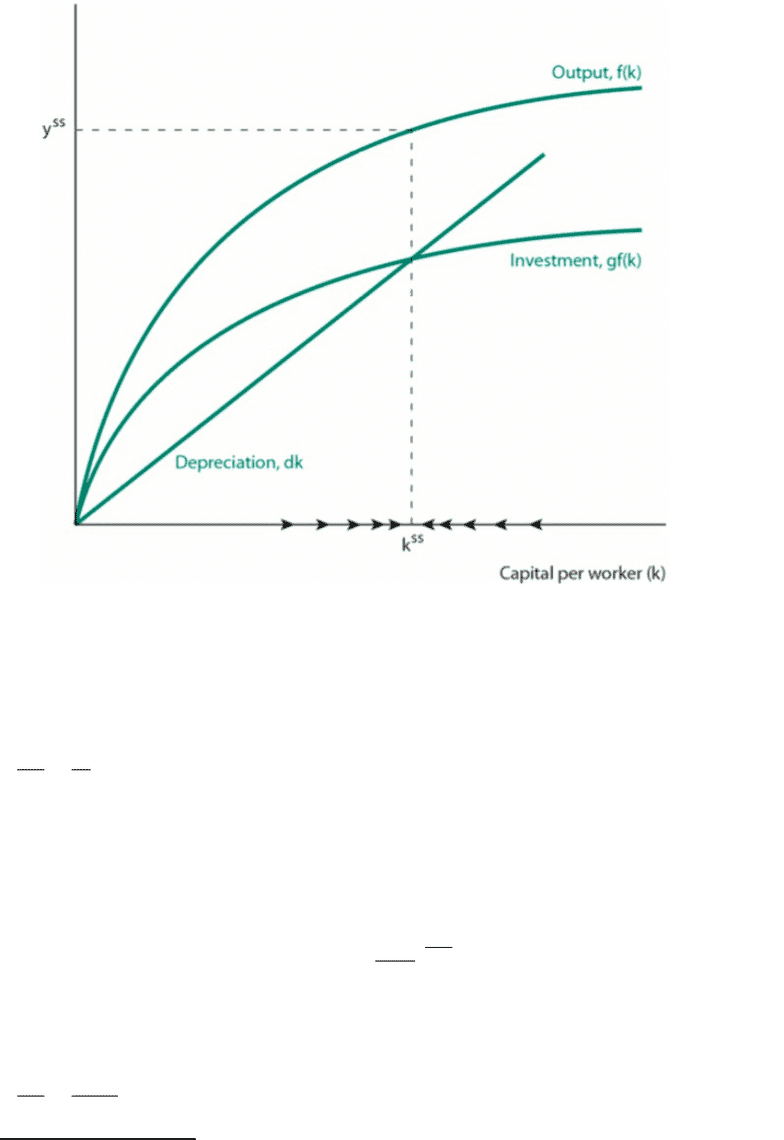# ECO2143 Chapter 1-7: Review for Midterm 1 on Growth

126 views9 pages
School
Department
Course
ProfessorEconomic growth is the change in GDP per capita.
There was virtually no growth in most countries before the industrial revolution.
Inequalities between countries are increasing.
Convergence is the idea that all countries converge towards the same GDP per
capita and that, as they approach this figure, their growth slows.
Capital is a physical object that let’s us work. It is
oProductive: its purpose is to create something.
oProduced: it comes from investment1. Since investment=savings (IS-LM),
this investment is symbolized by I=y, where is the saving rate.
oLimited.: only a limited amount of people can use it at the same time2.
oEarns a return: people are willing to pay to use it. It’s the incentive for its
production. For example, taxis are capital because the driver rents them. A
factory is also capital because it makes profit for the owner.
oDepreciates: It wears out. Symbolized by δ.
Human capital (labour) has all of these previously mentioned characteristics
except for limitation. Human capital also depreciates much slower than physical
capital and can only earn a return when there is work done
Productivity (A) is the effectiveness to which capital can be transformed into
output.
The two sources of growth are capital accumulation (increasing output per
worker) and productivity (increasing how much output is created for a fix level of
capital)
The production function, under its Cobb-Douglas form, is
F
(
K , N
)
=A Kα(h L)1α
, where A is productivity, K is capital, N is the number
of workers and is the capital shareɑ3. 4If we divide by N, output per worker is
then
F
(
K
N,1
)
=f
(
K
N
)
=y=A kαh1α
.
1 Land is not considered to be capital
2 An idea is not capital.
3 A number that gives the proportion of output that stems from
capital. In this course, we assume it is 1/3.
4 In the Cobb-Douglas model,
Output=Productivity × factors
1
Unlock document

This preview shows pages 1-3 of the document.
Unlock all 9 pages and 3 million more documents.

Already have an account? Log inNormal
Higher Productivity (A)
Lower productivity (A)
Capital per worker (k)
Output per worker (y)
The Solow Model links output per worker (y) and capital per worker (k):
k =γ yδk
Steady state occurs when capital doesn’t vary (∆k=0, or
γ y =δk
).
Replacing y by its Cobb-Douglas function, we have that capital at the
steady state is
γ A
(
kss
)
α=δ kss
Replacing this in the Cobb-Douglas function, we have that output at
the steady state equilibrium is
yss=A1/(1α)×
(
γ
δ
)
α
1α
In the previous model, we held labour output constant. However, if
we take in account the change in productivity output
A=A h1α
,
then this becomes
yss=h × A1/(1α)×
(
γ
δ
)
α
1α
2
Unlock document

This preview shows pages 1-3 of the document.
Unlock all 9 pages and 3 million more documents.

Already have an account? Log inoIf and δ are held constant, then, whatever k, output will always
tend towards the steady state. Therefore, the Solow model cannot
explain long-term growth (weakness).
oIf we have two countries i and j with the same productivity and
depreciation, then
yi
ss
yj
ss =
(
γi
γj
)
α/(1α)
oThe golden-rule level of capital is the that maximizes
consumption per worker5 in steady state. It’s the for which the
distance between the output curve and the depreciation curve is the
farthest.
oLet n be population growth. Then
yss=h × A1/(1α)×
(
γ
n+δ
)
α
1α
Therefore, if the saving rate and depreciation rate are the same
between two countries,
yi
ss
yj
ss =
(
δ+nj
δ+ni
)
α/(1α)
5 Consumption per worker :
c=f
(
kss
)
δ k ss
3
Unlock document

This preview shows pages 1-3 of the document.
Unlock all 9 pages and 3 million more documents.

Already have an account? Log in

# Get OneClass Notes+

Unlimited access to class notes and textbook notes.

75% OFF
\$8 USD/m
###### Monthly
\$30 USD/m
You will be charged \$96 USD upfront and auto renewed at the end of each cycle. You may cancel anytime under Payment Settings. For more information, see our Terms and Privacy.
Payments are encrypted using 256-bit SSL. Powered by Stripe.